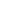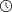Back to articles list- 6 minutes read

# How To Find the Closure of a Set of Attributes (With Examples)

Do you know what a closure of a set of attributes is? Do you know how to find it? This knowledge is essential for database normalization. In this article, learn what a closure of a set of attributes is and how to find it with real-world examples.

Finding the closure of a set of attributes is an important topic for relational databases. The closure of a set of attributes is taught in every university database class. And a common exam problem is, “Find the closure of this set of attributes given this set of functional dependencies.”Finding the closure of a set of attributes is needed for problems related to normalization. For example, you need to know how to compute the closure of a set of attributes to check if a set of attributes is a candidate key or a superkey. You also need this algorithm to decompose non-normal tables into normal forms.

## Closure of a Set of Attributes

The closure of a set of attributes X is the set of those attributes that can be functionally determined from X. The closure of X is denoted as X+.

When given a closure problem, you’ll have a set of functional dependencies over which to compute the closure and the set X for which to find the closure. A functional dependency A1, A2, …, An -> B in a table holds if two records that have the same value of attributes A1, A2, …, An also have the same value for attribute B.

The closure of X is the set of all attributes such that two records that have the same value of X also have the same value of X+.

## Real-World Examples

Database courses usually teach closures using abstract examples, and we’ll look at some abstract examples later. However, let’s first look at a real-world example, which is easier to understand.

Imagine we have a table `Course Editions`. The table contains information about editions of courses taught at a certain university.

Each year, each course can be taught by a different teacher. And each teacher has a date of birth. With the year and the date of birth, you can determine the age of the teacher at the time the course was taught.

(Note: This database is poorly designed. Don’t base your databases on this. We are only using this example to illustrate the concept of attribute closures.)

Course Editions

courseyearteacherdate_of_birthage
Databases2019Chris Cape1974-10-1245
Mathematics2019Daniel Parr1985-05-1734
Databases2020Jennifer Clock1990-06-0930

Here are the functional dependencies in this table:

• course, year -> teacher
• Given the course and year, you can determine the teacher who taught the course that year.
• teacher -> date_of_birth
• Given a teacher, you can determine the teacher’s date of birth.
• year, date_of_birth -> age
• Given the year and date of birth, you can determine the age of the teacher at the time the course was taught.

Now, let’s look at some of the attribute closures.

First, consider the closure of a set {year}, denoted {year}+. If I am given the year, what columns can I determine? I can for sure determine the year. So, the column year is an element of {year}+.

Are there any other columns that I can determine? The first functional dependency course, year -> teacher requires the course in addition to the year, so it doesn't add anything to {year}+. The functional dependency year, date_of_birth -> age requires the date of birth in addition to the year, so it doesn't add anything to {year}+ either.

So, {year}+ contains only one column, year, that is {year}+ = {year}.

Next, let’s look at {year, teacher}+. Given the year and teacher, what other columns can we determine? Well, as before, the year and teacher are given, so they are in {year, teacher}+.

If I know the teacher, I also know the date of birth because of the teacher -> `date_of_birth` functional dependency. So, date_of_birth is also in {year, teacher}+, and I know three columns: {year, teacher, date_of_birth}.

If I know the year and date of birth, I can also determine the age. Now, {year, teacher}+ has four columns {year, teacher, date_of_birth, age}.

I have used two of the three functional dependencies. I can’t use the remaining dependency, course, year -> teacher because I don’t know the course. Now that I have used all of the dependencies I can, {year, teacher}+ = {year, teacher, date_of_birth, age}.

## The Algorithm

The procedure shown in the previous example can be generalized to an algorithm. Assume we are given the set of functional dependencies FD and a set of attributes X. The algorithm is as follows:

1. Add the attributes contained in the attribute set X to the result set X+.
2. Add the attributes to the result set X+ which can be functionally determined from the attributes already contained in the result set.
3. Repeat step 2 until no more attributes can be added to the result set X+.

## Abstract Examples

Let’s take a look at some abstract examples. This is how the closure of a set of attributes would be presented in a class.

### Example 1

We are given the relation R(A, B, C, D, E). This means that the table R has five columns: A, B, C, D, and E. We are also given the set of functional dependencies: {A->B, B->C, C->D, D->E}.

What is {A}+?

• First, we add A to {A}+.
• What columns can be determined given A? We have A -> B, so we can determine B. Therefore, {A}+ is now {A, B}.
• What columns can be determined given A and B? We have B -> C in the functional dependencies, so we can determine C. Therefore, {A}+ is now {A, B, C}.
• Now, we have A, B, and C. What other columns can we determine? Well, we have C -> D, so we can add D to {A}+.
• Now, we have A, B, C, and D. Can we add anything else to it? Yes, since D -> E, we can add E to {A}+.
• We have used all of the columns in R and we have all used all functional dependencies. {A}+ = {A, B, C, D, E}.

### Example 2

Let’s look at another example. We are given R(A, B, C, D, E, F). The functional dependencies are {AB->C, BC->AD, D->E, CF->B}. What is {A, B}+?

• What columns can we determine, given A and B? We have AB -> C, so we can add C to {A, B}+.
• We now have A, B, and C. What other columns can we determine? We have BC -> AD. We already have A in {A, B}+, so we can add D.
• So, we now have A, B, C, and D. What else can we add? We have D -> E, so we can add E to {A, B}+.
• Now {A, B}+ is {A, B, C, D, E}. Can we add anything else? No. We have one more functional dependency in our set that we did not use: CF -> B. We can’t use this dependency because F is not in {A, B}+.
• Thus, {A, B}+ is {A, B, C, D, E}.